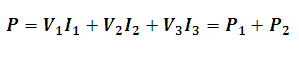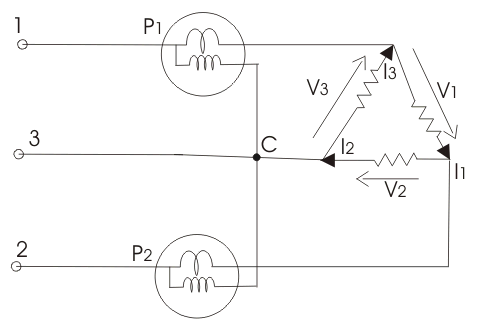# Three Phase Power Using Two Watt-meters in Delta Connection Load Calculator

This Calctown Calculator calculates the three phase power using two watt-meter method in a delta connection of load.

kW
kW

#### Result

kWwhere

P1 = reading of watt-meter 1

P2 = reading of watt-meter 2

V1 = 1st phase voltage

V2 = 2nd phase voltage

V3 = 3rd phase voltage

I1 = current in phase 1

I2 = current in phase 2

I3 = current in phase 3